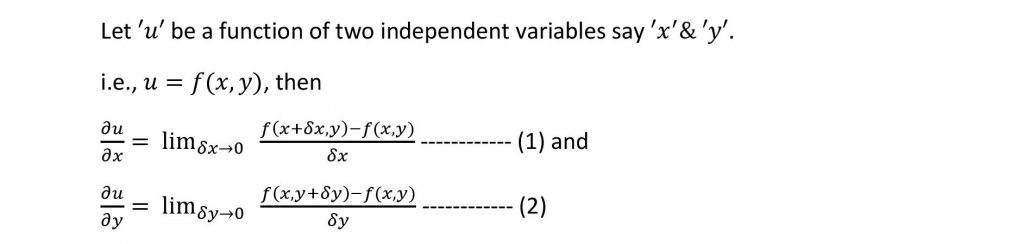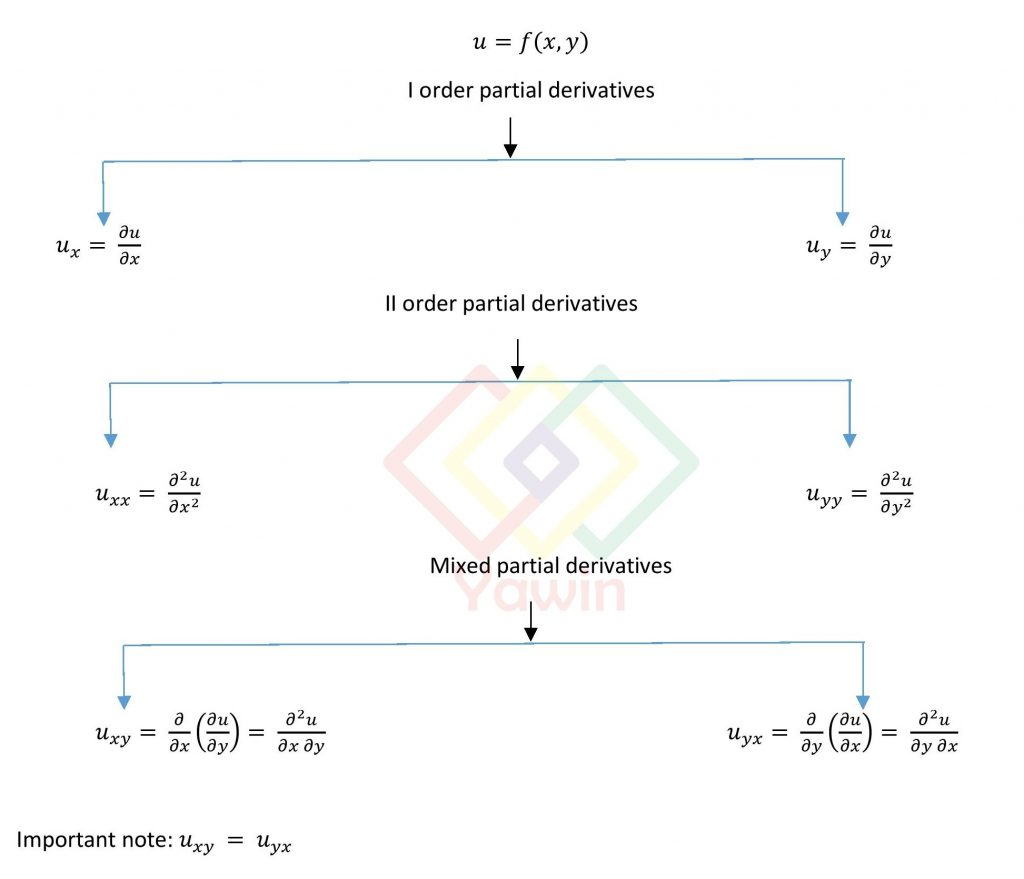Partial derivatives deals with the differentiation of a function of many independent variables.

## Partial derivatives Examples:

Area of rectangle depends on its length and breadth which is defined as A(l,b)

Volume of parallelepiped depends on its length, breadth and height which is defined as V(l,b,h)Where equation
(1) -> partial derivative of ‘u’ with respect to ‘x’, ‘y’ being a constant variable and
(2) -> partial derivative of ‘u’ with respect to ‘y’, ‘x’ being a constant variable.## Type 1: Direct partial derivatives

Symmetric Functions

A Function f(x,y) or f(x,y,z) is said to be symmetric if
f(x,y)=f(y,x) or
f(x,y,z)=f(z,y,x)=f(y,x,z)

## Type 2: Indirect partial derivatives

This is analogous to the concept of the differentiation of implicit functions in case of ordinary derivatives. Given a relation f(x,y,z)=c , we need to find the dependent variable and the associated independent variables based on the required partial derivatives.

Suppose we have x=f(u,v) and y=g(u,v) and if ∂u/∂x and ∂u/∂y are required then we have to express ‘u’ in terms of ‘x’ and ‘y’ as a single equation eliminating ‘v’ from the given relations.